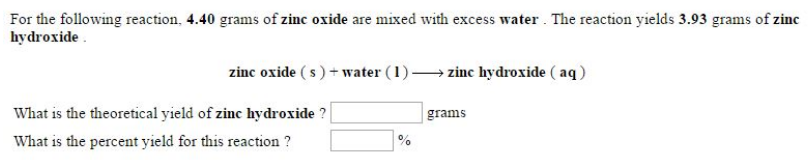# Problem: For the following reaction, 4.40 grains of zinc oxide are mixed with excess water. The reaction yields 3.93 grains of zinc hydroxide. zinc oxide (s) + water (l) → zinc hydroxide (aq) What is the theoretical yield of zinc hydroxide? What is the percent yield for this reaction?

###### FREE Expert Solution
81% (38 ratings)###### Problem Details

For the following reaction, 4.40 grains of zinc oxide are mixed with excess water. The reaction yields 3.93 grains of zinc hydroxide.

zinc oxide (s) + water (l) → zinc hydroxide (aq)

What is the theoretical yield of zinc hydroxide?

What is the percent yield for this reaction?